Browse by Topic

# Neural Networks: An Introduction

If you haven’t used machine learning, deep learning and neural networks yourself, you’ve almost certainly heard of them. You may be familiar with their commercial use in self-driving cars, image recognition, automatic text completion, text translation and other complex data analysis, but you can also train your own neural nets to accomplish tasks like identifying objects in images, generating sequences of text or segmenting pixels of an image. With the Wolfram Language, you can get started with machine learning and neural nets faster than you think. Since deep learning and neural networks are everywhere, let’s go ahead and explore what exactly they are and how you can start using them.

## What Exactly Are Neural Networks?

Neural networks are a programming approach that is inspired by the neurons in the human brain and that enables computers to learn from observational data, be it images, audio, text, labels, strings or numbers. They try to model some unknown function (for example,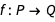) that maps this data to numbers or classes by recognizing patterns in the data. They are built from these components:

• Layers (to perform operations on these tensors, depending on the applications)
• Containers (to hold these operations in a sensible way)

Once the neural network is built from these components, it needs to be trained (in other words, optimized).

As you might have guessed, the optimization (or minimizing the “loss” of the network) is done through stochastic gradient descent in an iterative fashion. The inputs are fed to the net repeatedly; the error/loss is computed each time and is then used to update the model’s parameters using back propagation. Back propagation, or “back error propagation,” involves distributing the error computed during forward propagation back to the network’s layers.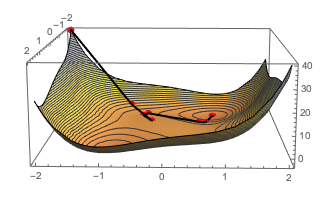## Understanding Encoders and Decoders

The input data provided in any form needs to be converted to numeric tensors. Here are a few examples of tensors and their corresponding ranks (dimensions):

• Rank 0 (scalars): 0.0
• Rank 1 (vectors): {0.0, 1.0}
• Rank 2 (matrices): {{1.,2.,3.} , {3., 2., 1.}}
• Rank-n tensors: {… {… {1., 2., 3.}…}…}

These examples provide valuable insight into how we can transform images into the corresponding ranked tensors: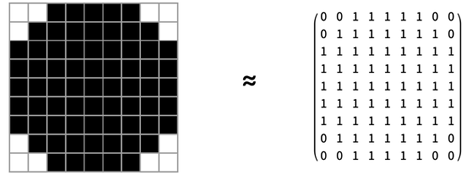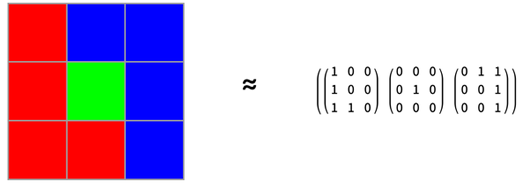### Encoder in Action

Take an image and convert it to tensors using a NetEncoder function, applied to images: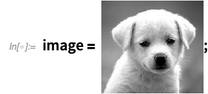✕ `image = CloudGet["https://wolfr.am/De49Ylam"];`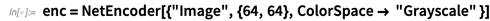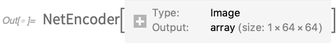✕ `enc = NetEncoder[{"Image", {64, 64}, ColorSpace -> "Grayscale" }]`

The variable encoded indeed contains a 64×64 matrix of a single dimension (corresponding to the grayscale ColorSpace). We can confirm that by looking at the dimensions of this array: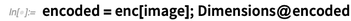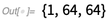✕ `encoded = enc[image]; Dimensions@encoded`

NetDecoder, on the other hand, can take a numeric tensor and convert it to the data type of your choice. Typically, one takes the output of the neural net and feeds it to the net decoder. Here, to illustrate the workings of a net decoder, let us simply feed back the “encoded” image to see if the decoder works as expected. Because we get our original image back in the specified 64×64 matrix, we have confirmation that it’s working: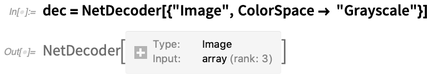✕ `dec = NetDecoder[{"Image", ColorSpace -> "Grayscale"}]`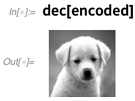✕ `dec[encoded]`

## Applying Layers

A neural network consists of “layers” through which information is processed from the input to the output tensor. Each layer is defined by its mathematical operation. So, mathematically, we can define a linear layer as an affine transformation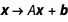, where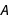is the “weight matrix” and the vector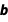is the “bias vector”: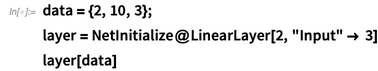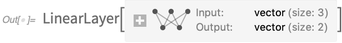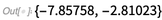✕ ```data = {2, 10, 3}; layer = NetInitialize@LinearLayer[2, "Input" -> 3] layer[data]```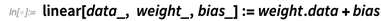✕ `linear[data_, weight_, bias_] := weight.data + bias`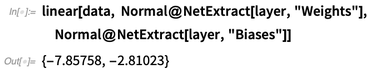✕ ```linear[data, Normal@NetExtract[layer, "Weights"], Normal@NetExtract[layer, "Biases"]]```

Here I’ve listed the various layers in the Wolfram Language. You can start exploring the layers, each of which is defined by its associated mathematical operation, to see what tasks they accomplish and how they do it.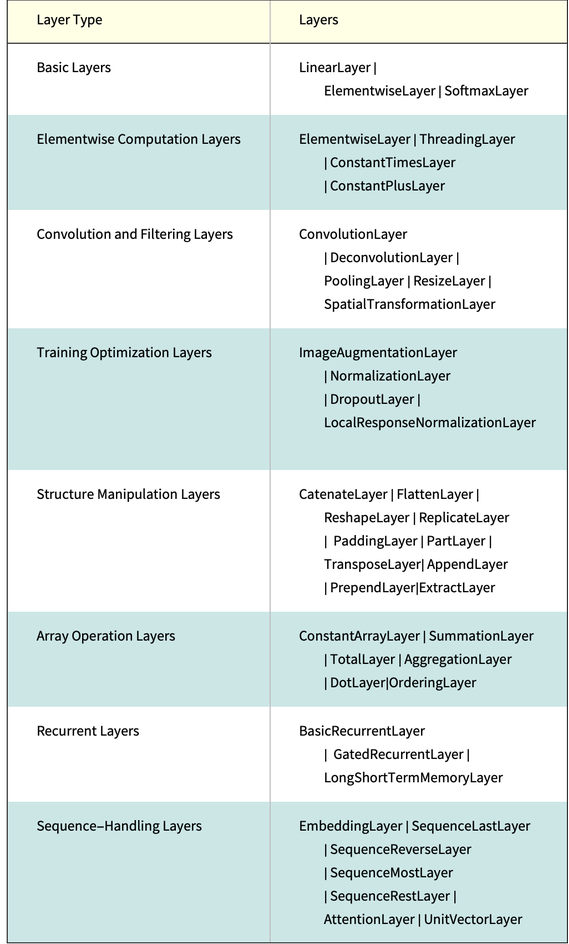Generally, most people use the following rule of thumb: “When you don’t know how to fine-tune your neural network, stack more layers.” So it makes us wonder: how do you stack them? The answer leads us to the third component mentioned earlier: containers.

## Using Containers

Once the data is converted into numeric tensors using NetEncoder and the layers are chosen for particular applications, they need to be “stitched” together using the containers. NetChain can be used to connect different layers in a chain-like fashion to create a neural net, while NetGraph can be used to connect different layers to create a graph, connecting these layers. Let’s try out some ways to use these containers to create networks.

Here we construct a chained network with nonlinear activation, which specifies the vector input of a specified size and produces vector outputs of size 3: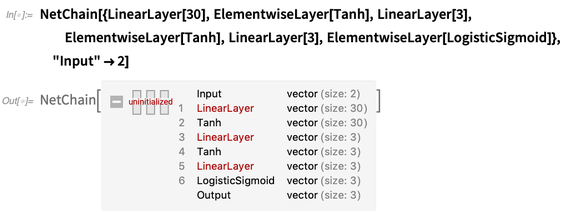✕ ```NetChain[{LinearLayer, ElementwiseLayer[Tanh], LinearLayer, ElementwiseLayer[Tanh], LinearLayer, ElementwiseLayer[LogisticSigmoid]}, "Input" -> 2]```

Combine two layers with NetGraph, using ThreadingLayer for the corresponding operation: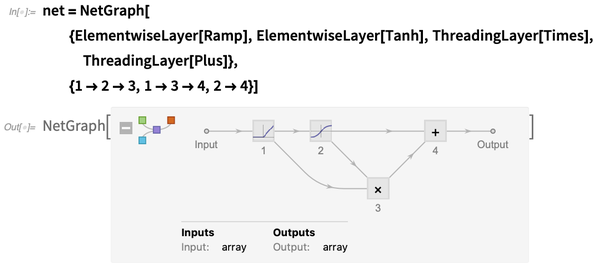✕ ```net = NetGraph[ {ElementwiseLayer[Ramp], ElementwiseLayer[Tanh], ThreadingLayer[Times], ThreadingLayer[Plus]}, {1 -> 2 -> 3, 1 -> 3 -> 4, 2 -> 4}]```

## The Problem of Overfitting

Stacking more layers brings us to a very serious problem of overfitting. When you keep stacking layers, you increase the number of tunable parameters in a network to thousands and even millions. The “deep” networks that are well suited to perform tasks like image classification, object detection and natural language processing are comprised of lots of stacked layers, and contain millions of parameters. When you have millions of parameters in your network, the network tends to “memorize” your data. In other words, the network will closely fit to your data, learn the eccentricities (or the “noise”) of your data and will not be able to generalize to other data. In an ideal world, where you have access to infinite data, the problem of overfitting would not arise. However, since we do not have access to infinite data (or the infinite training time required for infinite data), we need to make the best of what we have.

We divide our data into training, validation and test sets, keeping good enough percentages for the latter two. Finally, we can perform data augmentation: ImageAugmentationLayer automatically helps augment images to your dataset by performing random cropping and other operations. Next, we use various regularization techniques. Apart from the very familiar concepts of L1 and L2 regularization, which can be found as options for NetTrain, DropoutLayer can also be used to tackle the problem of overfitting. DropoutLayer sets the input elements to 0 with probability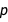during training, multiplying the remainder by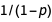and works as an effective and widely used regularization technique. Other methods like early stopping and multi-task learning can also be useful.

## Using the Wolfram Neural Net Repository

The current consensus in the neural net community is that building your own net architecture is unnecessary for the majority of neural net applications, and will usually hurt performance. Rather, adapting a pretrained net to your own problem is almost always a better approach in terms of performance. Luckily, this approach has the added benefit of being much easier to work with!

Thus, having a large neural net repository is absolutely key to being productive with the neural net framework, as it allows you to look for a net close to the problem you are solving, do minimal amounts of “surgery” on the net to adapt it to your specific problem and then train it.

The Wolfram Neural Net Repository gives Wolfram Language users easy access to the latest net architectures and pretrained nets, representing thousands of hours of computation time on powerful GPUs. The repository consists of publicly available models converted from other neural net frameworks (such as Caffe, Torch, MXNet, TensorFlow, etc.) into the Wolfram neural network format. In addition, we have trained a number of nets ourselves, which you can find in the Wolfram Neural Net Repository and the introductory blog. All the neural network models in the repository can be programmatically accessed via NetModel. Here’s a sample of 10 random models: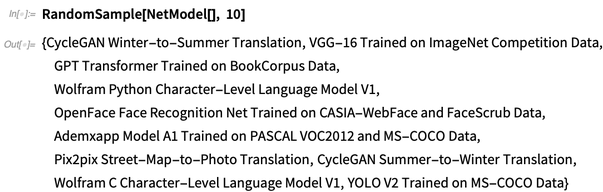✕ `RandomSample[NetModel[], 10]`

## Performing Network SurgeryLet’s look at an example of the net surgery process to solve the cat-versus-dog classification problem. First, obtain a net similar to our problem: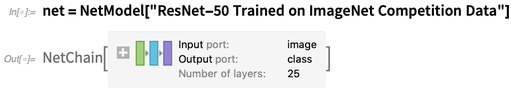✕ `net = NetModel["ResNet-50 Trained on ImageNet Competition Data"]`

The last two layers are specialized for the ImageNet classification task, and won’t be needed for our purposes. So we simply remove the last two layers using NetDrop: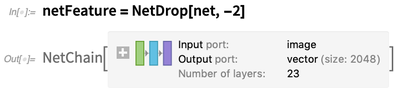✕ `netFeature = NetDrop[net, -2]`

Note that it is particularly easy to do “net surgery” in the Wolfram Language: nets are symbolic expressions that can be manipulated using a large set of surgery functions, such as NetTake, NetDrop, NetAppend, NetJoin, etc. Now we simply need to define a new NetChain that will classify an image as “dog” or “cat”: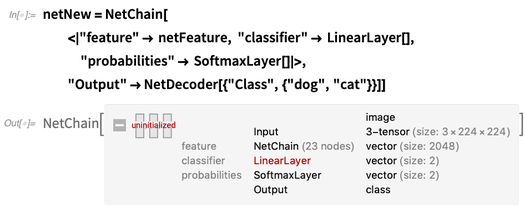✕ ```netNew = NetChain[ <|"feature" -> netFeature, "classifier" -> LinearLayer[], "probabilities" -> SoftmaxLayer[]|>, "Output" -> NetDecoder[{"Class", {"dog", "cat"}}]]```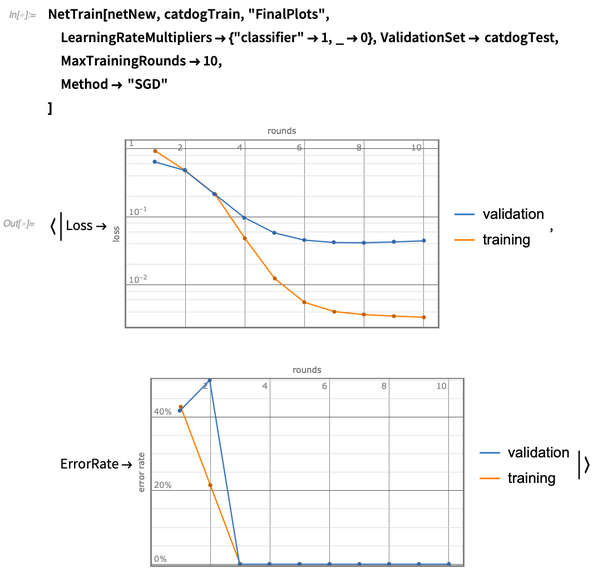✕ ```NetTrain[netNew, catdogTrain, "FinalPlots", LearningRateMultipliers -> {"classifier" -> 1, _ -> 0}, ValidationSet -> catdogTest, MaxTrainingRounds -> 10, Method -> "SGD" ]```

## Try Your Own Neural Network

Neural networks are data-driven algorithms, so the first step is to investigate your data thoroughly. Various statistical and visualization techniques can be used to see patterns and variations in the data. Once you have a better understanding of your data, decide on your network. The best bet is to start from networks that have been trained and validated by established researchers, or at least take inspiration from the various “building units” in them. A great place to start is the Wolfram Neural Net Repository, where you can play with various network surgery functions. Once you have created the architecture, start experimenting with various parameters, initializations and losses. It is absolutely okay to overfit at this stage! Finally, you can use regularization techniques in the original model or the ones discussed to generalize your model.

Visit the Wolfram Data Repository and Neural Net Repository for a combination of immediately useful resources for getting started.